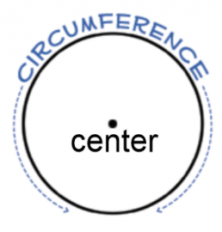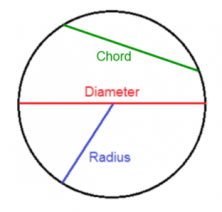Smartick is an advanced online program that teaches kids math and coding in only 15 min. a day

Aug26

# Geometric Shapes: Circle

We’ve already seen some of the main geometric shapes in earlier posts. In this post, we’re going to look more in-depth at the circle.

A circle is a geometrical shape that is made by drawing a curve that always has the same distance from the point that we refer to as the center.

In just a little bit, we’re going to learn about the different parts of a circle.

### Circumference:

The “length” of the circle is called the circumference.

The only difference between the circumference of the circle and a circle is that the circumference is a numerical value (sometimes with units), and a circle the actual figure that the circumference is measuring.

### Center:

The center of a circle is the point from which all points on the circle are the same distance.A radius is a segment drawn from the center of a circle to any point on a circle.

### Chord:

A chord is a segment drawn from one point on a circle to another point on a circle.

### Diameter:

A diameter is a chord that passes through the center of a circle. The diameter of a circle is the length of a diameter of the circle. The diameter is twice as long as the radius.